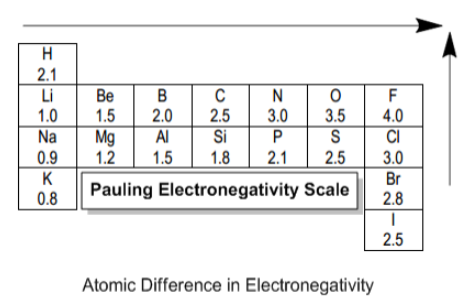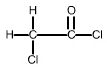# Problem: Analyze the polarity of each bond in the following organic compound. a) Which bond, other than the C-C bond, is the least polar in the molecule? C=O C-Cl C-H b) Which carbon atom has the most partial positive character? the left C the right C no difference

###### FREE Expert Solution

a. Analyzing the structure and atoms present:• Ranking for least to most electronegative is:

H < Cl < O

• Since H is the least electronegative, this will make the least polar bond

b.

• The atom with the most partial positive character is the C attached to the most EN atom
87% (127 ratings)###### Problem Details

Analyze the polarity of each bond in the following organic compound.a) Which bond, other than the C-C bond, is the least polar in the molecule?

C=O

C-Cl

C-H

b) Which carbon atom has the most partial positive character?

the left C

the right C

no difference